Area & Perimeter - 2

# Area & Perimeter - 2

Test Description

## 10 Questions MCQ Test Mathematics for Class 5 | Area & Perimeter - 2

Area & Perimeter - 2 for Class 5 2023 is part of Mathematics for Class 5 preparation. The Area & Perimeter - 2 questions and answers have been prepared according to the Class 5 exam syllabus.The Area & Perimeter - 2 MCQs are made for Class 5 2023 Exam. Find important definitions, questions, notes, meanings, examples, exercises, MCQs and online tests for Area & Perimeter - 2 below.
Solutions of Area & Perimeter - 2 questions in English are available as part of our Mathematics for Class 5 for Class 5 & Area & Perimeter - 2 solutions in Hindi for Mathematics for Class 5 course. Download more important topics, notes, lectures and mock test series for Class 5 Exam by signing up for free. Attempt Area & Perimeter - 2 | 10 questions in 10 minutes | Mock test for Class 5 preparation | Free important questions MCQ to study Mathematics for Class 5 for Class 5 Exam | Download free PDF with solutions
 1 Crore+ students have signed up on EduRev. Have you?
Area & Perimeter - 2 - Question 1

### Calculate the area of rectangle if one square represents an area of 1 cm2.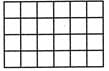Detailed Solution for Area & Perimeter - 2 - Question 1

There are 6×4=24 squares and each square has an area of = 1cm2. So, the area of rectangle =6×4×1=24cm2.

Area & Perimeter - 2 - Question 2

### Each side of square is of 10m. What will be the area of the square?

Detailed Solution for Area & Perimeter - 2 - Question 2

Area of square=side×side=10×10=100m2.

Area & Perimeter - 2 - Question 3

### Find the missing side in the figure given below if the perimeter of the figure is 28m?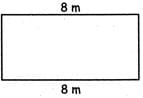Detailed Solution for Area & Perimeter - 2 - Question 3

Given figure is a rectangle. Also, given I = length = 8m Let b be the breadth of the rectangle. Perimeter of rectangle = 2(1 + b) = 28 ⇒   l + b = 28 / 2 ⇒   l + b = 14 ⇒ b = 14 ? 8 = 6m.

Area & Perimeter - 2 - Question 4

The length and breadth of a rectangle are (3a + 2) and (2a - 1). Which of the following represents its perimeter?

Detailed Solution for Area & Perimeter - 2 - Question 4

Perimeter = 2[3a + 2 + 2a - 1] = 2[5a + 1]

Area & Perimeter - 2 - Question 5

The area of a triangle whose base is 12 cm and the Height twice the base, is

Detailed Solution for Area & Perimeter - 2 - Question 5

Base ofΔ = 12 cm Height ofΔ=2×12=24cm Area ofΔ=1/2×12×24=144sq.cm.

Area & Perimeter - 2 - Question 6

The breadth of a rectangle is increased by 2 units. Its perimeter is now increased by?

Detailed Solution for Area & Perimeter - 2 - Question 6

We are given the following in the question:

The breadth of a rectangle is increased by 2 units.

Let l be the original length and b be the original breadth.

New breadth = b + 2

The perimeter of rectangle =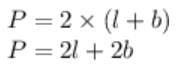The perimeter of new rectangle =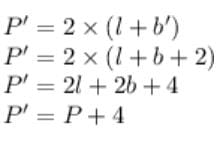Hence, the perimeter of rectangle increases by 4 units.

Area & Perimeter - 2 - Question 7

How many small cubes of side 2 cm can be put in a cubical box of side 6 cm?

Detailed Solution for Area & Perimeter - 2 - Question 7

Volume occupied by one cube of 2 cm = 2*2*2=8cm³

Volume occupied by one cube of 6 cm = 6*6*6=216cm³

No. of cubes of side 2 cm can be put in a cubical box of sides 6 cm = 216/8 = 27 cubes

Area & Perimeter - 2 - Question 8

Area of a square is:

Detailed Solution for Area & Perimeter - 2 - Question 8

side × side

Area & Perimeter - 2 - Question 9

Which of these figures represents the area of 24m2 if given that each block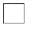=2m2

Detailed Solution for Area & Perimeter - 2 - Question 9

Given each block is of 2m2,so there should be 12 blocks in a figure to get an area of 24m2. In option (c), there are 2×6=12 blocks in figure.

Area & Perimeter - 2 - Question 10

Find area of unshaded region if each box = 3m2.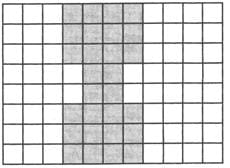Detailed Solution for Area & Perimeter - 2 - Question 10

No. of shaded boxes = 28, No. of unshaded boxes = 60 Area of 1 unshaded box =3m2 So, area of unshaded region = 60×3=180m2.

## Mathematics for Class 5

12 videos|55 docs|35 tests
 Use Code STAYHOME200 and get INR 200 additional OFF Use Coupon Code
Information about Area & Perimeter - 2 Page
In this test you can find the Exam questions for Area & Perimeter - 2 solved & explained in the simplest way possible. Besides giving Questions and answers for Area & Perimeter - 2, EduRev gives you an ample number of Online tests for practice

## Mathematics for Class 5

12 videos|55 docs|35 tests